! This post is also available in the following languages. Japanese, Korean

Multi-Threaded Parallel Processing for Physics Simulation in Cocos2d-x

Hello. My name is ST and I develop mobile games here at LINE. In this post I would like to talk about the multi-threaded parallel processing method we are using with Cocos2d-x, the leading mobile game engine. I will go into more detail about how we improved upon the existing single-thread structure and enhanced performance using multi-threaded physics calculation.

Multi-Threaded physics calculation parallel processing architecture

Before we move on to the multi-threaded physics calculation parallel processing structure, we should take a look at the existing single-thread Cocos2d-x update loop.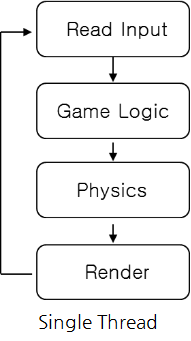[Figure 1] Original Cocos2d-x update loop

[Figure 1] depicts the existing update loop for Cocos2d-x. Once there is user input, it is sent through the game logic triggering a physics update which is then finally rendered. One thing to note here is that since it is based on a single-threaded system, rendering can only begin after the physics have been calculated. In other words, rendering is impossible before the physics calculation is complete. In cases where a high amount of calculation is required for physics simulation, rendering would be delayed resulting in low frame rates and graphical glitches.

The opposite can also occur. Too much load on rendering calculation can result in a delay in receiving user input, delaying the physics update process as well. All of this is due to the whole process being performed on a single loop. A single-threaded system would be adequate in cases where not much calculation is needed and where not many elements require processing. However, problems will occur if there is a heavy load.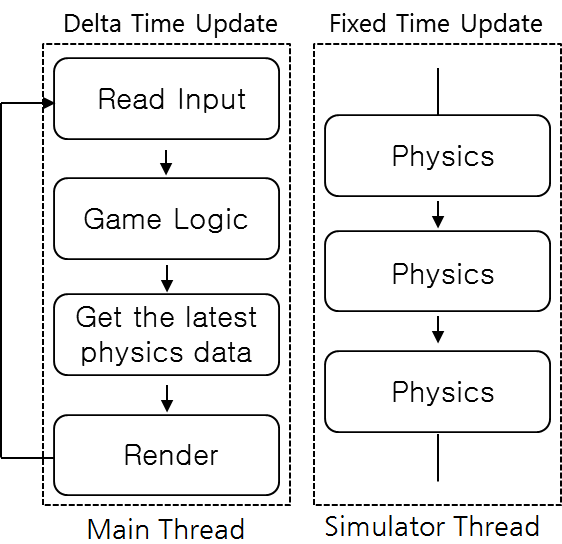[Figure 2] Proposed Cocos2d-x update loop

[Figure 2] is a blueprint of a multi-threaded physics calculation parallel processing loop running on Cocos2d-x. As you can see, the main difference is that all physics calculation is done on a separate thread while the main thread only receives and renders the latest calculated physics data.

With the original single-thread process, the update loop had to wait until physics calculation was complete before rendering the results. With this new structure, physics calculation is performed on a separate thread in parallel while the main thread renders the latest physics calculation sent from this thread. Even in situations where there is a heavy load on rendering, physics calculation can be performed in parallel while maintaining a stable number of ticks1.

The main thread uses Delta Time updating2 as it needs to display the results depending on time elapsed in the game, while the simulator thread uses Fixed Time updating3 to increase the accuracy of physics calculation. This parallel processing process, similar to the one found in the Unity engine, lets developers customize the Fixed Time value for more accurate physics simulation for their games.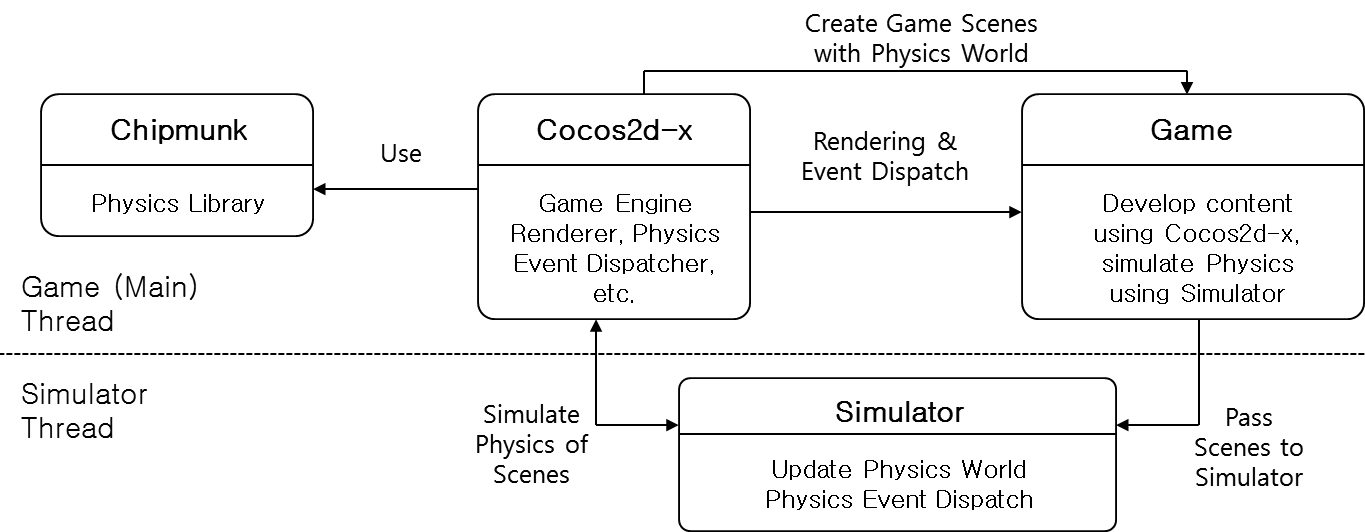[Figure 3] System architecture

[Figure 3] depicts the multi-threaded architecture applied to Cocos2d-x, now capable of processing physics calculation in parallel. The following 4 modules were used for this system.

• Cocos2d-x
• Chipmunk Physics Library
• Game
• Simulator

Cocos2d-x comes equipped with a 2D physics library named Chipmunk. Game uses Cocos2d-x to create the needed Scenes among other content. Simulator can access Cocos2d-x through the received Scene data, and then acquire control of the dynamic physics calculation capabilities of Chipmunk through Cocos2d-x. Cocos2d-x, Chipmunk, and Game are run on the main thread while Simulator runs on a separate thread. In other words, Game and physics calculation are processed in parallel.

1: A tick occurs when the update function is called. Tick count is the number of times the update function is called. The time between the last update and the most recent update is known as Delta Time, a smaller Delta Time causes a shorter update interval which in turn generates more update ticks.

2: A Delta Time update performs updates based on the time gap between the current frame and the last. Hence known as a Delta Time (1/FPS) update.

3: A Fixed Time update performs updates based on units of fixed time.

In order to perform multi-threaded physics calculation, a well-designed physics simulator is crucial.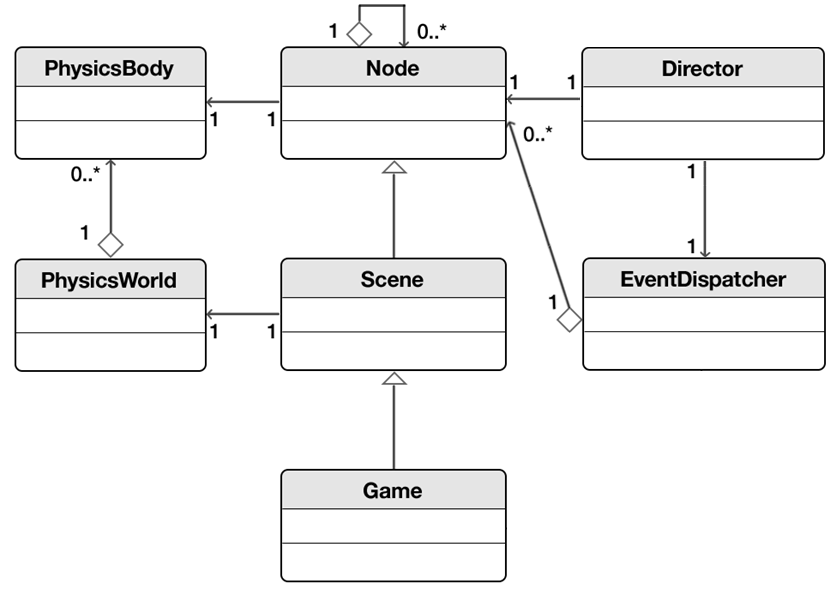[Figure 4] Original single-thread Cocos2d-x physics structure

[Figure 4] depicts the original physics structure of Cocos2d-x. As you can see, Node and PhysicsBody are connected one-to-one and so is Scene and PhysicsWorld. Scene can have several child Nodes while PhysicsWorld can also have more than one PhysicsBody. Scene, the topmost object containing scenes of the game, is connected one-to-one with PhysicsWorld, the topmost object containing physics. A global EventDispatcher variable is used for event handling.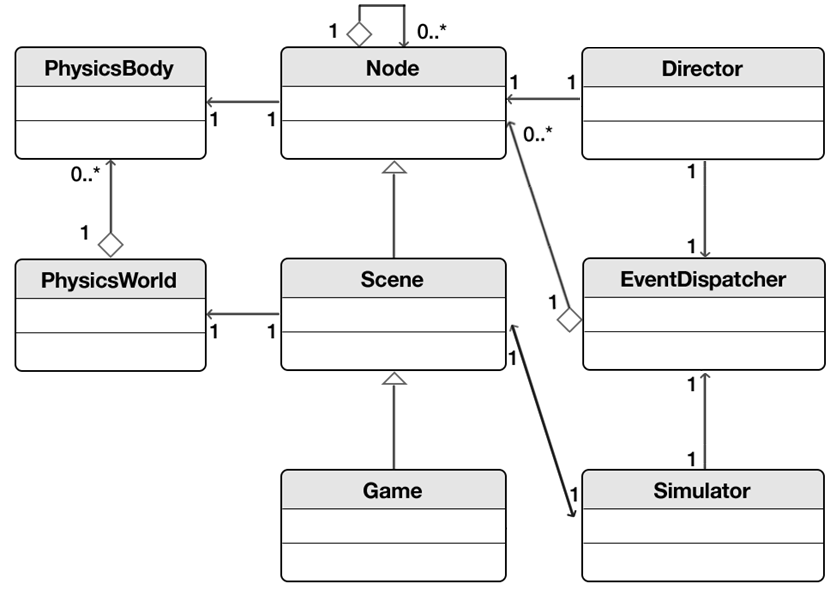[Figure 5] Newly designed multi-threaded physics structure for Cocos2d-x

How can we enable a multi-threaded system from this current structure? If you look closely at [Figure 5], you will see that Simulator has been added. Simulator has a Scene object and an EventDispatcher object. Simulator accesses PhysicsWorld through Scene, and then gains access to the physics data in PhysicsBody through PhysicsWorld. PhysicsBody is a class that wraps cpBody, a physics Rigid Body object that is provided by Chipmunk. The PhysicsBody object has direct access to the data in the Chipmunk physics library in Cocos2d-x. In other words, in order to enable parallel physics calculation in Cocos2d-x you must add synchronization logic not only to the source code of Cocos2d-x but also on the source code of Chipmunk.

To set up synchronization I downloaded the Chipmunk physics library source code and added a mutex synchronization logic to the section where the cpBody value changes, and then added synchronization logic to PhysicsBody on Cocos2d-x as it also uses cpBody. TryLock will determine the status of a read lock, and the system will render the most recent value without waiting for the lock to be released. However, as Cocos2d-x handles events with a single EventDispatcher variable, if the simulator thread attempts to access the main thread when an EventDispatcher is triggered it can cause errors.

There are two ways to solve this problem. The first is to set up a critical zone using mutex, and the second is to generate an additional independent EventDispatcher variable on the simulator thread. Mutex was used sparingly for the sake of performance, and the EventDispatcher variable was declared on Simulator so that collision events could be handled directly and independently on the simulator thread without conflicting with the main thread. Finally, a build of Chipmunk with synchronization logic added to the source code was used to create the libchipmunk.a library file, which was then used to build a modified version Cocos2d-x source code, creating the libgame.so file. As a result, the game can be run using the libgame.so file.

Testing environment

I tested the newly designed multi-threaded system to see how much the physics simulation was improved over the single-threaded system. Below are the conditions of the test. The device used was an Apple iPhone 5S. The software used was Cocos2d-x version 3.6 with Chipmunk version 2.2.2.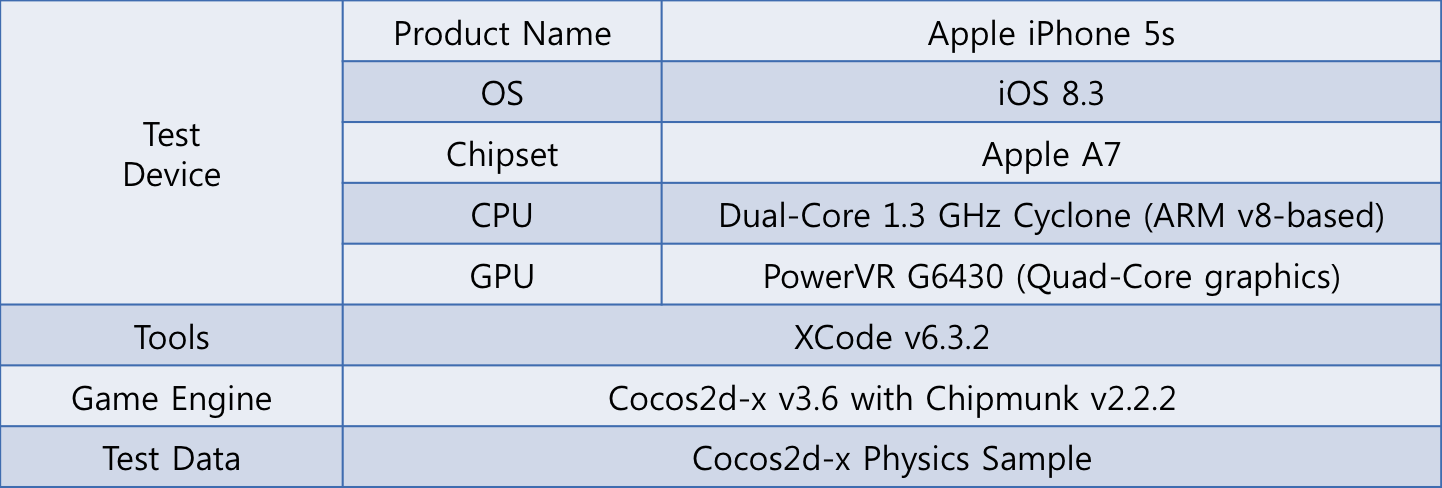[Table 1] Testing environment

Synchronization and event handling test

I created a test program to see if synchronization and event handling still works as intended after implementing the multi-threaded parallel processing system for physics calculation. The program was based on the default Contact test included with Cocos2d-x.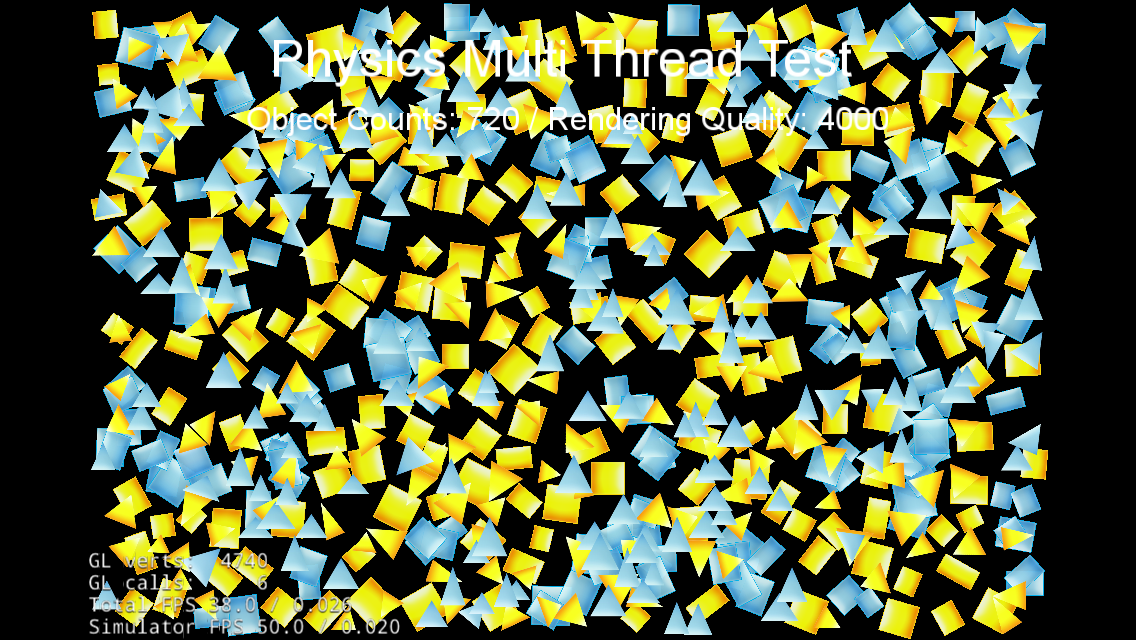[Figure 6] Test program

[Figure 6] is a screenshot from the test program after it has generated several triangular and square objects. These objects were set to bounce off when they collide with each other or with the invisible walls surrounding the screen. To test synchronization and event handling, a callback function was set up so that objects would turn green when they collide.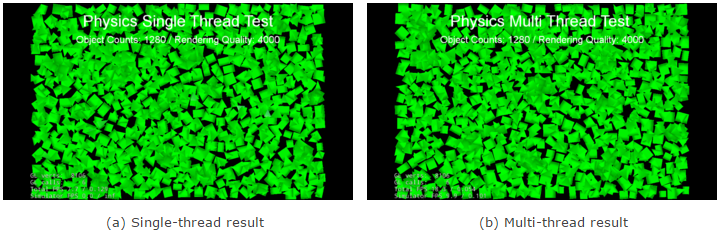[Figure 7] Physics simulation result

The tests for single-thread and multi-thread were both done under identical conditions. The results can be seen through [Figure 7] (a) and (b). For both single-thread and multi-thread scenarios, the objects all changed into green. The objects colliding and bouncing off each other proves that synchronization occurs, and their colors changing to green after a collision proves that event handling is working as intended by allowing the callback function to properly change the objects to green. Now that we have confirmed that synchronization and event handling are working properly, we can move on to testing performance.

Performance test results

This performance test will let us know how much our multi-threaded physics calculation improves performance compared to a single-threaded system.

Testing the effect of overhead on game performance

There are moments during game development where the game experiences overhead, causing it to be more sluggish than usual. I tested how having a multi-threaded physics calculation affects the performance of the game. As overhead in a game causes more load on the CPU’s calculation capabilities, we defined the amount of overhead by multiplying the matrix several times.

MAX_UPDATE_COUNT = 300000
for i ← 1 to MAX_UPDATE_COUNT
do dstMatrix ← dstMatrix * srcMatrix

This way we can output the amount of overhead in a quantifiable value using the MAX_UPDATE_COUNT variable.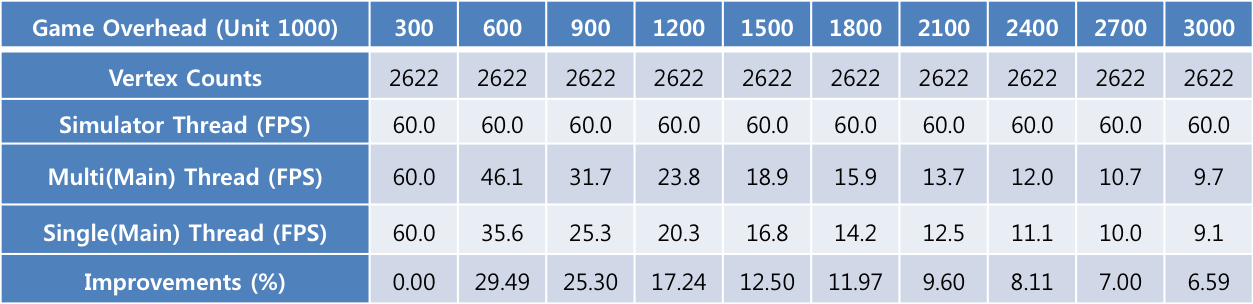[Table 2] Performance affected by game overhead

What this tells us is that regardless of the physics calculation method, the main thread will experience overhead and performance will decrease. However, as physics calculation occurs on a different thread (the simulator thread) it is not affected by game overhead, and the performance was 60 FPS regardless of whether overhead was 300,000 or 3,000,000.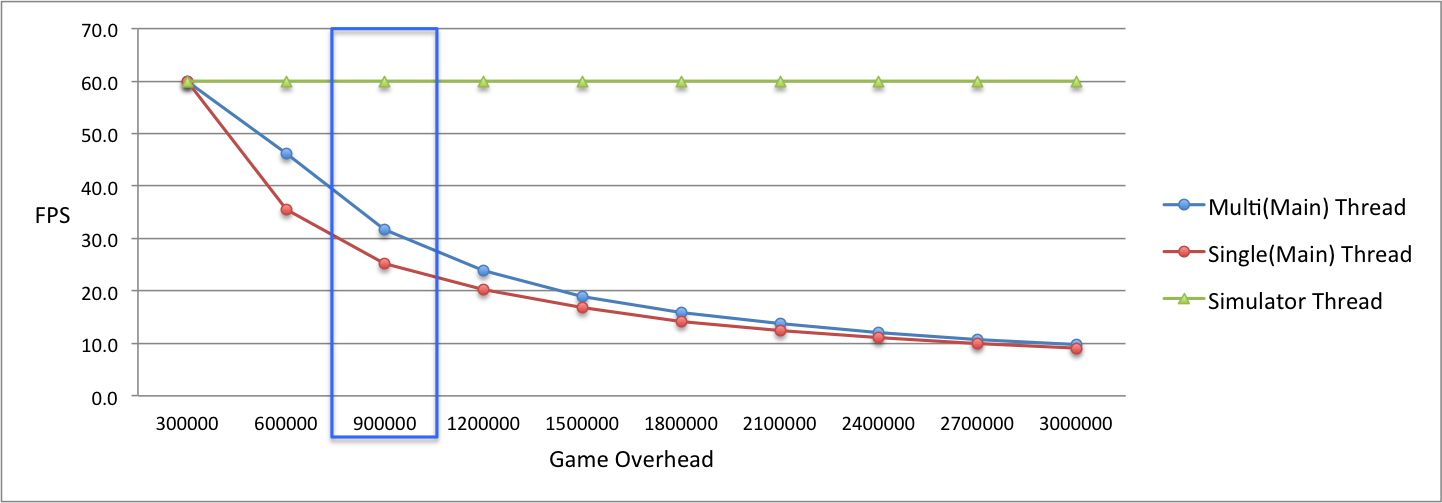[Figure 8] Performance affected by game overhead

[Figure 8] is a graph depicting the impact of overhead on game performance. We can see on the curves for single-thread and multi-thread main threads (blue and red) that the performance drop is less severe on the main thread of the multi-threaded system. While performance drops more severely on a single-threaded system due to physics calculation and rendering occurring on a single loop, a multi-threaded system suffers less thanks to a separate simulator thread handling the physics calculation.

The results above are from a stress test. But which of these values could be applied to a real-world scenario? The section with 900,000 overhead where the game is rendering at 30 FPS would be one such example (the section highlighted in blue on [Figure 8]). The frame rate in this section was 25.3 FPS on single-thread, 31.7 FPS on multi-thread, and 60 FPS on the simulator thread.

When physics calculation is updated in Delta Time, physics were calculated and rendered in approximately 0.0395 seconds (25.3 FPS) on a single-thread while it took 0.0167 seconds (60 FPS) to calculate physics and 0.0315 seconds (31.7 FPS) to render on a multi-thread. Since a multi-threaded system can calculate physics and collision check in 2.4 times more detail while rendering at a higher frame rate, the difference in quality is noticeable. When tested in Fixed Time at 0.0167 seconds, a single-threaded system steps4 at a value of 0.0167 every 0.0395 seconds (25.3 FPS), processing and simulating collision.

A multi-threaded system on the other hand, steps at a value of 0.0167 every 0.0167 seconds (60 FPS). While the simulated steps along with the accuracy of the simulation were identical, the multi-threaded system had 2.4 times more ticks, making simulation faster than on a single-threaded system.

4: A step is practically the same as an update, which means that physics simulation occurs. They are called steps because the updates are performed step by step.

Testing the effects of overhead on physics calculation performance

There are times during development where the slow physics calculation brings down the performance of the game, more so than because of graphical issues or an issue in the game logic. We tested to observe what the effects of overhead on physics calculation would be.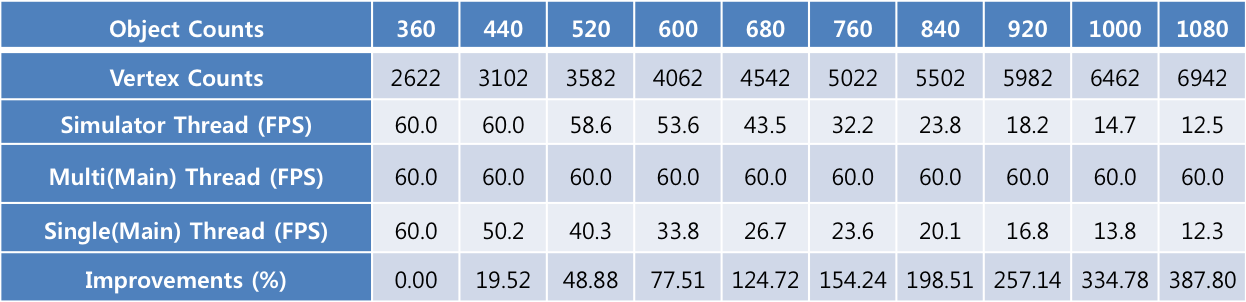[Table 3] Performance affected by physics calculation overhead

Since there was overhead on physics calculation, this caused performance loss on both the single-threaded system and the simulator thread of the multi-threaded system. But the main thread of the multi-threaded system was still able to render at 60 FPS even with 1080 objects, as its game logic and rendering operates separately from the simulator thread.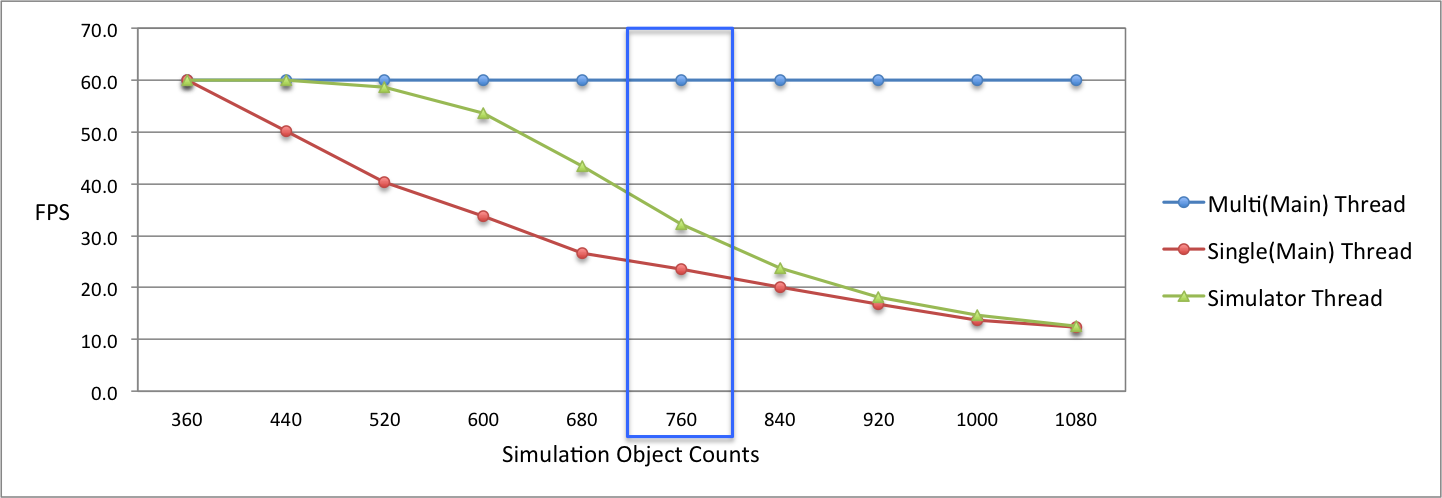[Figure 9] Performance affected by physics calculation overhead

The results above are from a stress test. But which of these values could be applied to a real-world scenario? The section with 760 objects where the game is rendering at 30 FPS would be one such example (the section highlighted in blue on [Figure 9]).　The single-threaded system was recorded at 23.6 FPS, the main thread of the multi-threaded system was 60 FPS, and the simulator thread was 32.2 FPS. When physics calculation is updated in Delta Time, physics were calculated and rendered in approximately 0.0424 seconds (23.6 FPS) on a single-thread while it took 0.0311 seconds (32.2 FPS) to calculate physics and 0.0167 seconds (60 FPS) to render on a multi-thread.

Since a multi-threaded system can calculate physics every 0.0311 seconds on a separate thread and render the simulated physics on the main thread every 0.0167 seconds, the quality of the collision checking is much higher. When tested in Fixed Time at 0.0167 seconds, a single-threaded system steps at a value of 0.0167 every 0.0424 seconds (23.6 FPS), processing and simulating collision. A multi-threaded system on the other hand, steps at a value of 0.0167 every 0.0311 seconds (32.2 FPS) while also rendering the calculated values every 0.0167 seconds (60 FPS). The multi-threaded is already slightly faster than the single-threaded system, but it also renders objects much smoother as its rendering FPS is 2.5 times higher.

Collision check accuracy test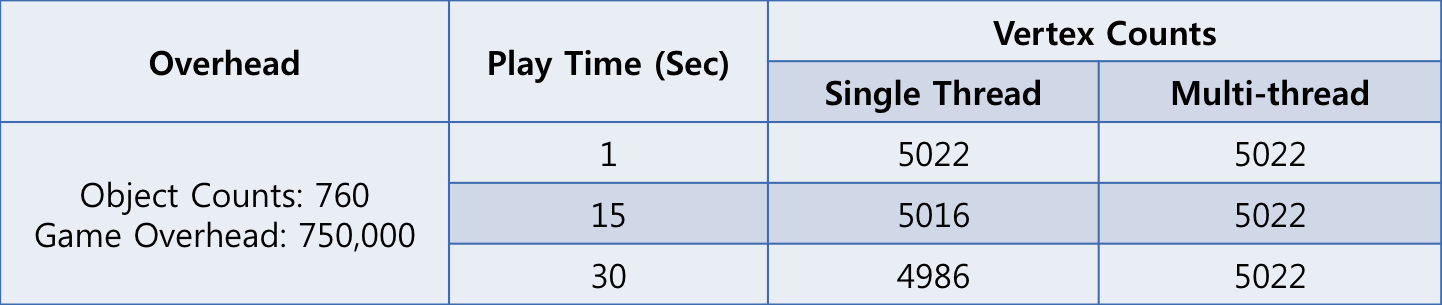[Table 4] Collision check accuracy test